Function Repository Resource:

# IntervalGraph

Construct the intersection graph of intervals

Contributed by: Wolfram Staff (original content by Sriram V. Pemmaraju and Steven S. Skiena)
 ResourceFunction["IntervalGraph"][{interval1,interval2,…}] constructs the interval Graph defined by the intervali.

## Details and Options

ResourceFunction["IntervalGraph"][{interval1,interval2,}] returns an undirected Graph with a vertex for each intervali and edges between vertices whose intervals intersect.
The intervali must be defined on the real line.
ResourceFunction["IntervalGraph"] accepts the options of Graph.

## Examples

### Basic Examples (1)

The interval Graph of three intervals, two of which are overlapping:

 In:=Out=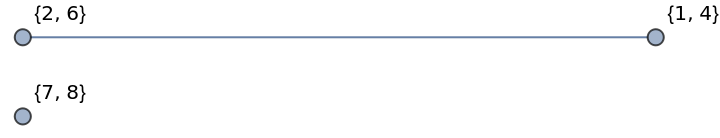### Properties and Relations (2)

Graphs returned by IntervalGraph are chordal and perfect:

 In:=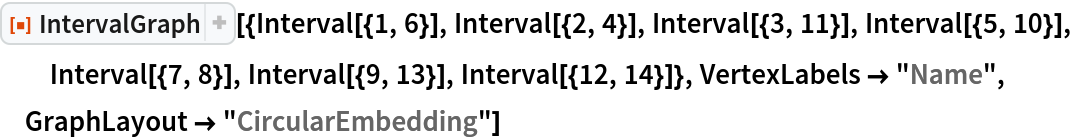Out=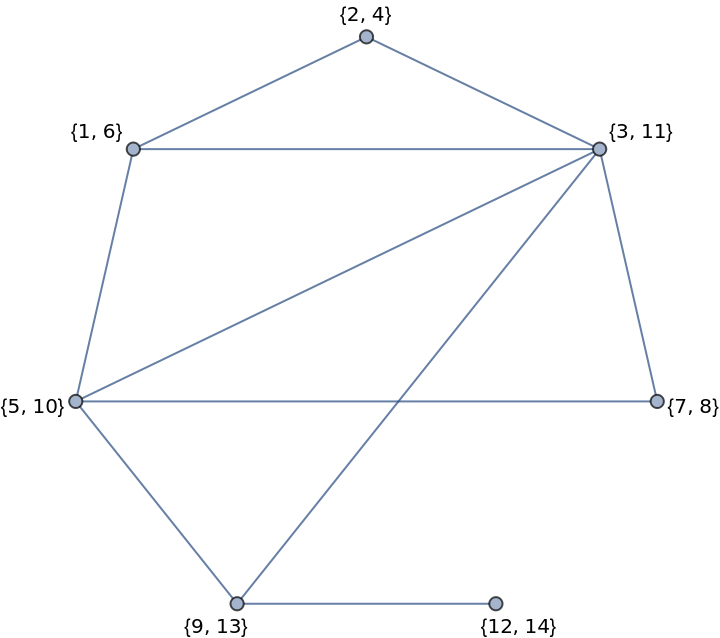In:=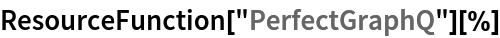Out=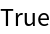Star graphs can be constructed as interval graphs:

 In:=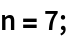In:=Out=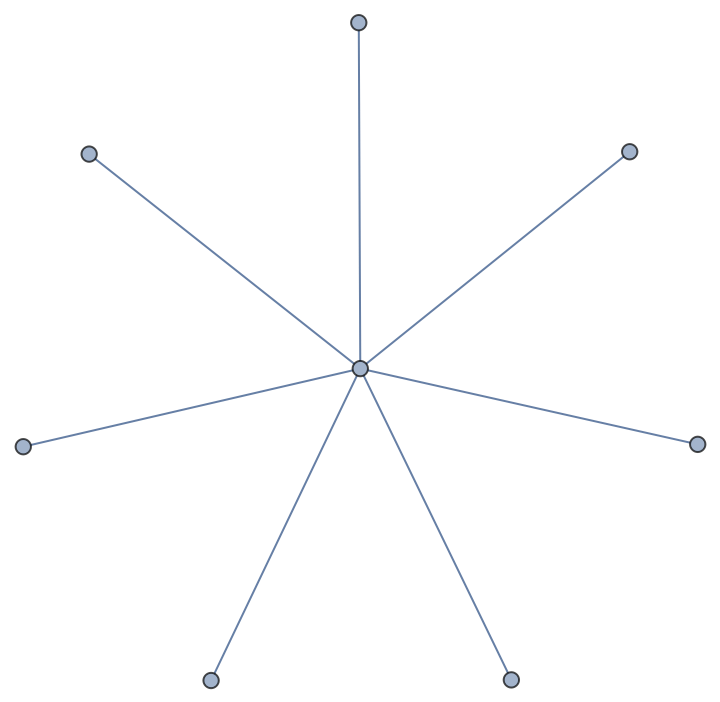In:=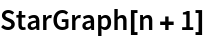Out=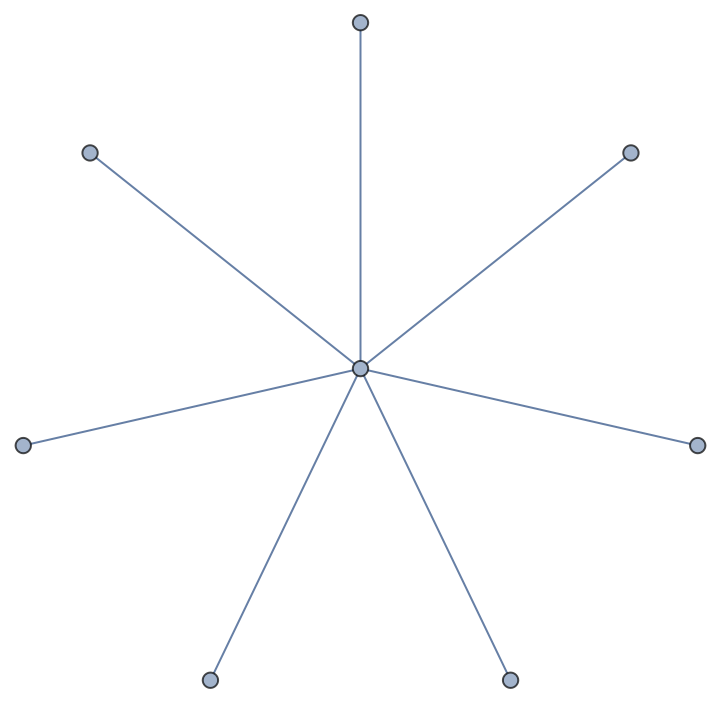## Version History

• 1.0.0 – 22 July 2020